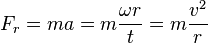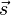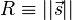# Centripetal force facts for kids

Kids Encyclopedia Facts

Centripetal force is an accelerating force that acts on any body that revolves around a centre. This force contributes to keeping the body in rotation. This force is always directed towards the centre.

The opposite force (by Isaac Newton's third law of motion) is called centrifugal force. This is the force that acts on the body in a direction away from the centre, which contributes to making the body try to fly away. When you hold a rope with a heavy object attached to it, and rotate it around, the rope becomes tight and keeps the body from flying away. This is caused by centripetal force.

An example is a roller coaster which uses centripetal force to accelerate the carts so they will keep going in a circular motion. Even if an object changes direction but maintains at a constant speed it still counts as acceleration.$F_r = ma =m \frac{\omega r}{t} = m \frac{v^2}{r}$

## Geometric proof for uniform circular motion

In the figure to the right we define the displacement vector$\vec s$ to represent motion in a circle. The magnitude of$\vec s$ is denoted as$R\equiv ||\vec s||$ and represents the radius of the particle's orbit.

## Images for kidsCentripetal force Facts for Kids. Kiddle Encyclopedia.